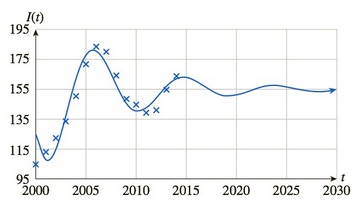Chapter 10.1, Problem 59EFinite Mathematics and Applied Cal...

7th Edition
Stefan Waner + 1 other
ISBN: 9781337274203

Solutions

Chapter
SectionFinite Mathematics and Applied Cal...

7th Edition
Stefan Waner + 1 other
ISBN: 9781337274203
Textbook Problem

Home Prices The following graph shows the values of the home price index12 for 2000–2014 together with a mathematical model I extrapolating the data:Estimate and interpret lim t → + ∞ I ( t ) ,

To determine

The value of limt+I(t) from the graph which shows the values of the home price index for years 20002014 and also interpret the answer.Explanation

Given information:

The graph which shows the values of the home price index for years 20002014 is as follows:

From the graph which shows the values of the home price index for years 20002014 with mathematical model I extrapolating the data, the value of limt+I(t) can be calculated.

Consider the expression, limt+I(t)

For calculatin

Still sussing out bartleby?

Check out a sample textbook solution.

See a sample solution

The Solution to Your Study Problems

Bartleby provides explanations to thousands of textbook problems written by our experts, many with advanced degrees!

Get Started

1. Find the first 4 terms of the sequence with nth term

Mathematical Applications for the Management, Life, and Social Sciences

An iterated integral for the volume of the solid shown is:

Study Guide for Stewart's Multivariable Calculus, 8th

Which is the graph of ?

Study Guide for Stewart's Single Variable Calculus: Early Transcendentals, 8th